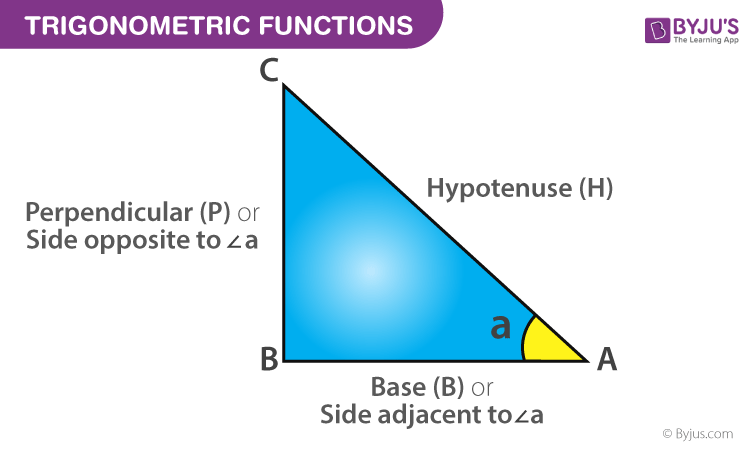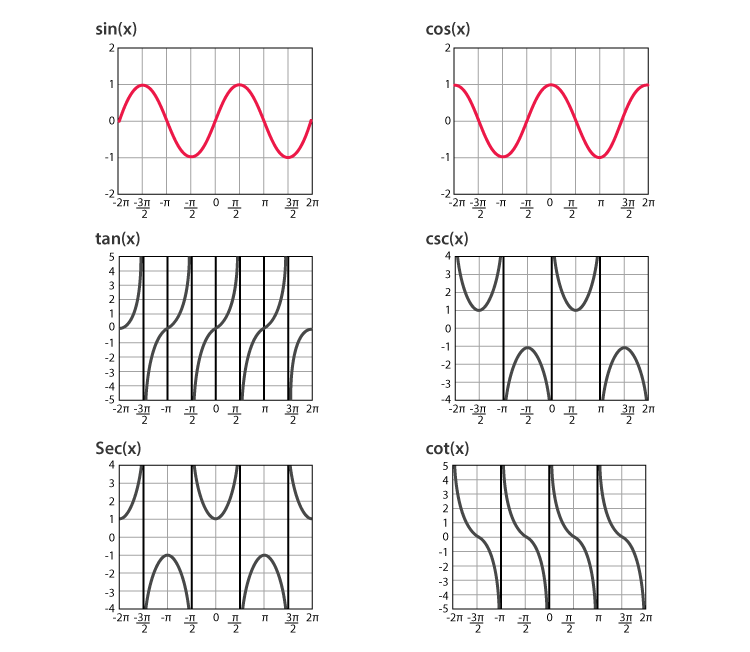# Trigonometric Functions

Trigonometric functions are also known as Circular Functions can be simply defined as the functions of an angle of a triangle. It means that the relationship between the angles and sides of a triangle are given by these trig functions. The basic trigonometric functions are sine, cosine, tangent, cotangent, secant and cosecant. Also, read trigonometric identities here.

There are a number of trigonometric formulas and identities that denotes the relation between the functions and help to find the angles of the triangle. All these trigonometric functions with their formula are explained here elaborately, to make them understand to the readers.

Also, you will come across the table where the value of these ratios is mentioned for some particular degrees.  And based on this table you will be able to solve many trigonometric examples and problems.

## Six Trigonometric Functions

The angles of sine, cosine, and tangent are the primary classification of functions of trigonometry. And the three functions which are cotangent, secant and cosecant can be derived from the primary functions. Basically, the other three functions are often used as compared to the primary trigonometric functions. Consider the following diagram as a reference for an explanation of these three primary functions. This diagram can be referred to as the sin-cos-tan triangle. We usually define trigonometry with the help of the right-angled triangle.

##### Sine Function

Sine function of an angle is the ratio between the opposite side length to that of the hypotenuse. From the above diagram, the value of sin will be:

• Sin a =Opposite/Hypotenuse = CB/CA

### Cos Function

Cos of an angle is the ratio of the length of the adjacent side to the length of the hypotenuse. From the above diagram, the cos function will be derived as follows.

• Cos a = Adjacent/Hypotenuse = AB/CA

### Tan Function

The tangent function is the ratio of the length of the opposite side to that of the adjacent side. It should be noted that the tan can also be represented in terms of sine and cos as their ratio. From the diagram taken above, the tan function will be the following.

• Tan a = Opposite/Adjacent = CB/BA

Also, in terms of sine and cos, tan can be represented as:

Tan a = sin a/cos a

### Secant, Cosecant and Cotangent Functions

Secant, cosecant (csc) and cotangent are the three additional functions which are derived from the primary functions of sine, cos, and tan. The reciprocal of sine, cos, and tan are cosecant (csc), secant (sec), and cotangent (cot) respectively. The formula of each of these functions are given as:

• Sec a = 1/(cos a) =  Hypotenuse/Adjacent = CA/AB
• Cosec a = 1/(sin a) = Hypotenuse/Opposite = CA/CB
• cot a = 1/(tan a) = Adjacent/Opposite = BA/CB

NoteInverse trigonometric functions are used to obtain an angle from any of the angle’s trigonometric ratios. Basically, inverses of the sine, cosine, tangent, cotangent, secant, and cosecant functions are represented as arcsine, arccosine, arctangent, arc cotangent, arc secant, and arc cosecant.

## Formulas

Let us discuss the formulas given in the table below for functions of trigonometric ratios(sine, cosine, tangent, cotangent, secant and cosecant) for a right-angled triangle.

 Formulas for Angle θ Reciprocal Identities sin θ = Opposite Side/Hypotenuse sin θ = 1/cosec θ cos θ = Adjacent Side/Hypotenuse cos θ = 1/sec θ tan θ = Opposite Side/Adjacent tan θ = 1/cot θ cot θ = Adjacent Side/Opposite cot θ = 1/tan θ sec θ = Hypotenuse/Adjacent Side sec θ = 1/cos θ cosec θ = Hypotenuse/Opposite cosec θ = 1/sin θ

## Identities

Below are the identities related to trig functions.

### Even and Odd functions

The cos and sec functions are even functions; the rest other functions are odd functions.

sin(-x) = -sin x

cos(-x) = cos x

tan(-x) = – tan x

cot(-x) = -cot x

csc(-x) = -csc x

sec(-x) = sec x

### Periodic Functions

The trig functions are the periodic functions. The smallest periodic cycle is 2π but for tangent and the cotangent it is π.

sin(x+2nπ) = sin x

cos(x+2nπ) = cos x

tan(x+nπ) = tan x

cot(x+nπ) = cot x

csc(x+2nπ) = csc x

sec(x+2nπ) = sec x

Where n is any integer.

### Pythagorean Identities

When the Pythagoras theorem is expressed in the form of trigonometry functions, it is said to be Pythagorean identity. There are majorly three identities:

• sin2 x + cos2 x = 1    [Very Important]
• 1+tan2 x = sec2 x
• cosec2 x = 1 + cot2 x

These three identities are of great importance in Mathematics, as most of the trigonometry questions are prepared in exams based on them. Therefore, students should memorise these identities to solve such problems easily.

### Sum and Difference Identities

• sin(x+y) = sin(x).cos(y)+cos(x).sin(y)
• sin(x–y) = sin(x).cos(y)–cos(x).sin(y)
• cos(x+y) = cosx.cosy–sinx.siny
• cos(x–y) = cosx.cosy+sinx.siny
• tan(x+y) = [tan(x)+tan(y)]/[1-tan(x)tan(y)]
• tan(x-y) = [tan(x)-tan(y)]/[1+tan(x)tan(y)]

## Video Lessons

### Trigonometric Functions Session 1### Trigonometric Functions Session 2### Trigonometric Functions Session 3### Trigonometric Functions-Session 4### Trigonometric Functions-Session 5### Trigonometric Functions Session 6## Table

The trigonometric ratio table for six functions like Sin, Cos, Tan, Cosec, Sec, Cot, are:

 Trigonometric Ratios/ angle= θ in degrees 0 ° 30 ° 45 ° 60 ° 90 ° Sin θ 0 1/2 1/√2 √3/2 1 Cos θ 1 √3/2 1/√2 1/2 0 Tan θ 0 1/√3 1 √3 ∞ Cosec θ ∞ 2 √2 2/√3 1 Sec θ 1 2/√3 √2 2 ∞ Cot θ ∞ √3 1 1/√3 0

## Graphs

By now we have known the formulas and values for different angles for all the trigonometric functions. Let us see here the graphs of all the six trigonometric functions to understand the alteration with respect to a time interval.

Before we see the graph, let us see the domain and range of each function, which is to be graphed in XY plane.

 Function Definition Domain Range Sine Function y=sin x x ∈ R − 1 ≤ sin x ≤ 1 Cosine Function y = cos x x ∈ R − 1 ≤ cos x ≤ 1 Tangent Function y = tan x x ∈ R , x≠(2k+1)π/2, − ∞ < tan x < ∞ Cotangent Function y = cot x x ∈ R , x ≠ k π − ∞ < cot x < ∞ Secant Function y = sec x x ∈ R , x ≠ ( 2 k + 1 ) π / 2 sec x ∈  ( − ∞ , − 1 ] ∪ [ 1 , ∞ ) Cosecant Function y = csc x x ∈ R , x ≠ k π csc x ∈  ( − ∞ , − 1 ] ∪ [ 1 , ∞ )

Here is the graph for all the functions based on their respective domain and range.## How to Solve Trigonometric Functions?

### Solving Trigonometric Functions## Solved Examples on Trigonometric Functions

Example 1: Find the values of Sin 45°, Cos 60° and Tan 60°.

Solution: Using the trigonometric table, we have

Sin 45° = 1/√2

Cos 60° = 1/2

Tan 60° = √3

Example 2: Evaluate Sin 105° degrees.

Solution: Sin 105° can be written as sin (60° + 45°) which is similar to sin (A + B).

We know that, the formula for sin (A + B) = sin A × cos B + cos A × sin B

Therefore, sin 105° = sin (60° + 45°) = sin 60° × cos 45° + cos 60° × sin 45°

= √3/2 × 1/√2 + 1/2 × 1/√2

= √3/2√2 + 1/2√2
= (√3+1)/2√2

Example 3: A boy sees a bird sitting on a tree at an angle of elevation of 20°. If a boy is standing 10 miles away from the tree, at what height bird is sitting?

Solution: Consider ABC a right triangle, A is a bird’s location, B = tree is touching the ground and C = boy’s location.

So BC 10 miles, angle C = 20° and let AB = x miles

We know, tan C = opposite side/adjacent side

tan(20°) = x/10

or x = 10 × tan(20°)

or x = 10 × 0.36 = 3.6

Bird is sitting at the height of 3.6 miles from the ground.

### Trigonometry Related Articles for Class 11 and 12

Register with  BYJU’S to get more such maths-related articles in a simple and detailed way. Also, register at BYJU’S to get access to 1000+ hours of engaging video lessons for different subjects and classes.

## Frequently Asked Questions on Trigonometric Functions

Q1

### What are the six trigonometric functions?

The six trigonometric functions are Sine, Cosine, Tangent, Secant, Cosecant and Cotangent.
Q2

### What is the use of trigonometric functions?

In geometry, trigonometric functions are used to find the unknown angle or side of a right-angled triangle.
Q3

### What are the three basic trigonometric functions?

The three basic trigonometry functions are Sine, Cosine and Tangent.
Q4

### What is the formula for trigonometry functions?

If θ is an angle of a right-angled triangle, then the trigonometry functions are given by:
sin θ = Opposite Side of angle θ/Hypotenuse
cos θ = Adjacent Side of angle θ/Hypotenuse
tan θ = Opposite Side of angle θ/Adjacent
cot θ = Adjacent Side of angle θ/Opposite
sec θ = Hypotenuse/Adjacent Side of angle θ
cosec θ = Hypotenuse/Opposite Side of angle θ
Q5

### What is the value of sin θ, cos θ and tan θ, if θ=30 degrees?

If θ = 30 degrees, then,
Sin θ = sin 30 = ½
Cos θ = cos 30 = √3/2
Tan θ = tan 30 = 1/√3
Q6

### What is the range of sin, cos and tan function in a periodic graph?

Sine Function: y = sinx; Domain: x ∈ R & Range: − 1 ≤ sin x ≤ 1
Cos Function: y = cos x; Domain: x ∈ R & Range: − 1 ≤ cos x ≤ 1
Tan Function: y=tan x; Domain: x ∈ R , x≠(2k+1)π/2 & Range: − ∞ < tan x < ∞
Test your knowledge on Trigonometric Functions